# how to schedule warps?

In paper , authors measure pipeline latency on several graphic card and reports in table 1.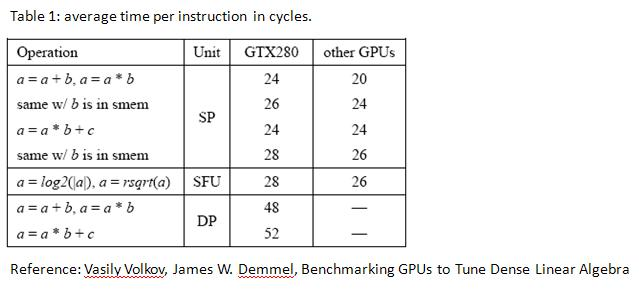the table shows that register-to-register MAD (multiply-and-add) instruction runs at 24 cycles.

and authors argue “24 cycle latency may be hidden by running simultaneously 6 warps (or 192 threads) per SM”.

this match description section 5.1.2.6 n programming guide,

"Generally, accessing a register is zero extra clock cycles per instruction, but delays may occur due to register read-after-write dependencies and register memory bank conflicts.

The delays introduced by read-after-write dependencies can be ignored as soon as there are at least 192 active threads per multiprocessor to hide them"

My question is : how does scheduler dispatch warps in a SM? Two methods,

Method 1 : Warp occupies SPs till memory-access instruction is executed.

Method 2 : Each warp execute one instruction in turn.

In section 4.1 of programming guide, it says â€œEvery instruction issue time, the SIMT unit selects a warp that is ready to execute and issues the next instruction to the active threads of the warpâ€.

It seems that hardware supports method 2.

I take an example to show method 1 and method 2.

Example : execute three instructions S1, S2 and S3 in turn

``````S1 : a <-- a * b + c; // register read-after-write dependence

S2 : a <-- a * b + c; // register read-after-write dependence

S3 : odata[index] <-- a;// read operation
``````

we show Gatt chart of method 1 in figure 1 and Gatt chart of method 2 in figure 2.

figure 1,

figure 2,

Reference:  Vasily Volkov, James W. Demmel, Benchmarking GPUs to Tune Dense Linear Algebra

Ehh, yes that is how it works according to all the material that is available on the issue. Each tick another warp is selected to run.

What you call method 1 would be pretty pointless ;)

yes, method 2 can hide piepline latency if 6 warps are issued, moreover

if we invoke 192 threads (6 warps) per block, then Gatt chart is independent of whether read-after-write hazard occurs or not.

Although method 2 is right, but it is tedious to draw Gatt chart under method 2.

I donâ€™t adopt method 2 to draw Gatt chart when compare bandwidth between “float” and “double” in the thread

In that topic, I donâ€™t use pipeline latency but calibrate â€œindex computationâ€ via Block-wise test harness provided by SPWorley.

in the thread http://forums.nvidia.com/index.php?showtopic=103046 , @SPWorley uses one block of 192 threads to calibrate

“how many clocks the operation takes”.

following code is kernel of calibration.

``````#define ITEST(num) \

ikernel<itest_ ## num, EVALLOOPS> <<<1,192>>>(12345, d_result); \

cudaMemcpy( &h_result, d_result, sizeof(int), cudaMemcpyDeviceToHost); \

printf("I" #num " %4.1lf %s\n", \

8*(h_result-ibase+1.0)/(192*EVALLOOPS*UNROLLCOUNT), \

itest_ ## num ().describe());
``````

@SPWorley’s code uses

(1) 192 threads to hide pipeline latency and

(2) unroll large loop

Question: what is relationship between pipeline latency and SPWorley uses one block of 192 threads to calibrate “how many clocks the operation takes”.

first suppose we want to evaluate operation S1 ( a <-- a * b + c ), then we must do S1 large times, say M times.

``````for i = 1: M

S1 : a <-- a * b + c; // register read-after-write dependence

end

S2 : a <-- a * b + c; // register read-after-write dependence
``````

Then it is easy to plot Gatt chart of above code, just modify Gatt chart in figure 2, repeat operation S1 M times , see figure 3.

figure 3,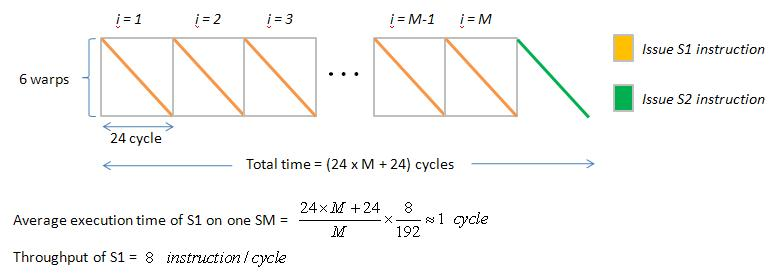if M is large enough, then average execution time of S1 on one SM is about 1 cycle.

(when all 8 SP executes S1 simultaneously, it only needs 1 cycle to complete S1)

similarly if we want to calibrate operation “S1 + S2” in the following code,

``````for i = 1: M

S1 : a  <-- a * b + c; // register read-after-write dependence

S2 : a  <-- a * b + c; // register read-after-write dependence

end

S3 : odata[index]  <-- a; // write operation
``````

then Gatt chart is figure 4. average execution time of S1+S2 on one SM is about 2 cycle

figure 4,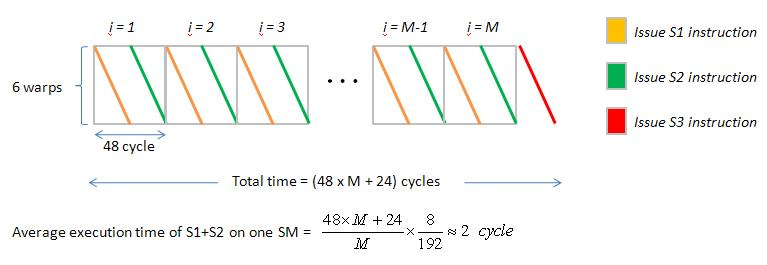Average execution time of S1 on one SM = 1 cycle, this means that one warp needs 4 cycle to execute S1 instruction

in average sense. We define method 3 as method 1 but with average execution time of instructions.

Then Gatt chart of following code is figure 5

``````//Example : execute three instructions S1, S2 and S3 in turn

S1 : a <-- a * b + c; // register read-after-write dependence

S2 : a <-- a * b + c; // register read-after-write dependence

S3 : odata[index] <-- a; // write operation

S4 : a <--  a * b + c;
``````

figure 5,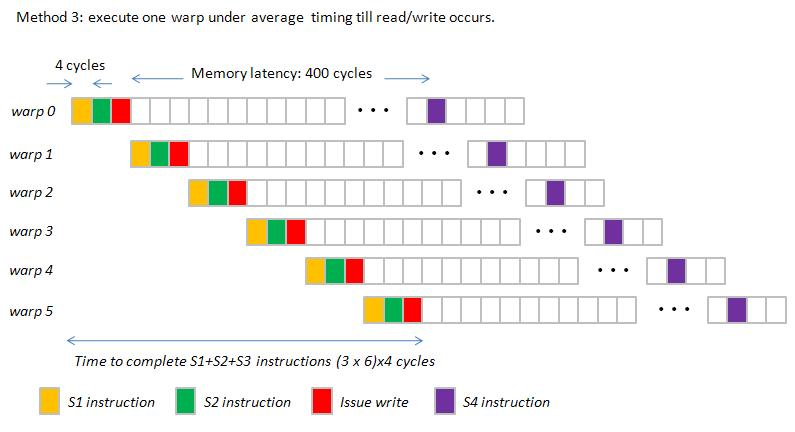however if we use method 2, then Gatt chart is figure 6

figure 6,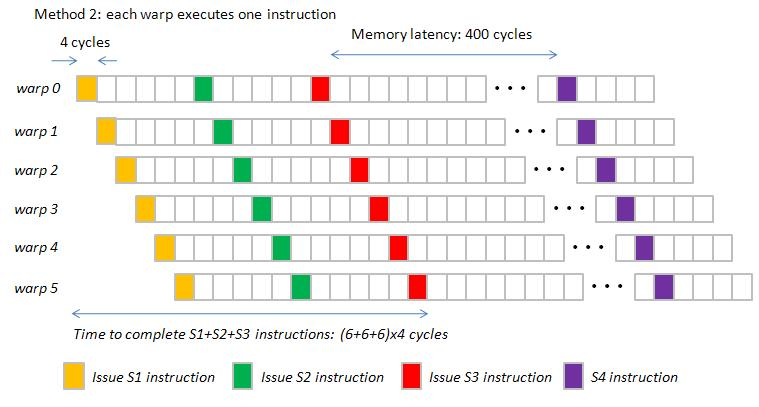Observation: difference between method 2 and method 3

(1) Method 3 hides “index computation” in memory latency while method 2 hide “index computation” in pipeline latency.

(2) Space between two read/write operation (red rectangle) is larger in method 3.

However critical timing of method 2 and method 3 are the same, so we can use method 3 to plot Gatt chart.

To sum up, I think that it is reasonable to draw Gatt chart by method 3, which is more simple.